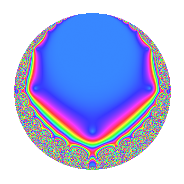# Properties

 Label 7.7.b.bLevel 7 Weight 7 Character orbit 7.b Analytic conductor 1.610 Analytic rank 0 Dimension 2 CM No Inner twists 2

# Related objects

## Newspace parameters

 Level: $$N$$ = $$7$$ Weight: $$k$$ = $$7$$ Character orbit: $$[\chi]$$ = 7.b (of order $$2$$ and degree $$1$$)

## Newform invariants

 Self dual: No Analytic conductor: $$1.61037858534$$ Analytic rank: $$0$$ Dimension: $$2$$ Coefficient field: $$\Q(\sqrt{-510})$$ Coefficient ring: $$\Z[a_1, a_2, a_3]$$ Coefficient ring index: $$2$$ Sato-Tate group: $\mathrm{SU}(2)[C_{2}]$

## $q$-expansion

Coefficients of the $$q$$-expansion are expressed in terms of $$\beta = 2\sqrt{-510}$$. We also show the integral $$q$$-expansion of the trace form.

 $$f(q)$$ $$=$$ $$q$$ $$-8 q^{2}$$ $$+ \beta q^{3}$$ $$-\beta q^{5}$$ $$-8 \beta q^{6}$$ $$+ ( 133 + 7 \beta ) q^{7}$$ $$+ 512 q^{8}$$ $$-1311 q^{9}$$ $$+O(q^{10})$$ $$q$$ $$-8 q^{2}$$ $$+ \beta q^{3}$$ $$-\beta q^{5}$$ $$-8 \beta q^{6}$$ $$+ ( 133 + 7 \beta ) q^{7}$$ $$+ 512 q^{8}$$ $$-1311 q^{9}$$ $$+ 8 \beta q^{10}$$ $$+ 874 q^{11}$$ $$+ 49 \beta q^{13}$$ $$+ ( -1064 - 56 \beta ) q^{14}$$ $$+ 2040 q^{15}$$ $$-4096 q^{16}$$ $$-132 \beta q^{17}$$ $$+ 10488 q^{18}$$ $$+ 69 \beta q^{19}$$ $$+ ( -14280 + 133 \beta ) q^{21}$$ $$-6992 q^{22}$$ $$+ 4738 q^{23}$$ $$+ 512 \beta q^{24}$$ $$+ 13585 q^{25}$$ $$-392 \beta q^{26}$$ $$-582 \beta q^{27}$$ $$+ 11146 q^{29}$$ $$-16320 q^{30}$$ $$-608 \beta q^{31}$$ $$+ 874 \beta q^{33}$$ $$+ 1056 \beta q^{34}$$ $$+ ( 14280 - 133 \beta ) q^{35}$$ $$+ 3002 q^{37}$$ $$-552 \beta q^{38}$$ $$-99960 q^{39}$$ $$-512 \beta q^{40}$$ $$+ 1274 \beta q^{41}$$ $$+ ( 114240 - 1064 \beta ) q^{42}$$ $$+ 31418 q^{43}$$ $$+ 1311 \beta q^{45}$$ $$-37904 q^{46}$$ $$-1604 \beta q^{47}$$ $$-4096 \beta q^{48}$$ $$+ ( -82271 + 1862 \beta ) q^{49}$$ $$-108680 q^{50}$$ $$+ 269280 q^{51}$$ $$-76406 q^{53}$$ $$+ 4656 \beta q^{54}$$ $$-874 \beta q^{55}$$ $$+ ( 68096 + 3584 \beta ) q^{56}$$ $$-140760 q^{57}$$ $$-89168 q^{58}$$ $$+ 2507 \beta q^{59}$$ $$-6091 \beta q^{61}$$ $$+ 4864 \beta q^{62}$$ $$+ ( -174363 - 9177 \beta ) q^{63}$$ $$+ 262144 q^{64}$$ $$+ 99960 q^{65}$$ $$-6992 \beta q^{66}$$ $$+ 495242 q^{67}$$ $$+ 4738 \beta q^{69}$$ $$+ ( -114240 + 1064 \beta ) q^{70}$$ $$-184406 q^{71}$$ $$-671232 q^{72}$$ $$-1350 \beta q^{73}$$ $$-24016 q^{74}$$ $$+ 13585 \beta q^{75}$$ $$+ ( 116242 + 6118 \beta ) q^{77}$$ $$+ 799680 q^{78}$$ $$-534934 q^{79}$$ $$+ 4096 \beta q^{80}$$ $$+ 231561 q^{81}$$ $$-10192 \beta q^{82}$$ $$-15827 \beta q^{83}$$ $$-269280 q^{85}$$ $$-251344 q^{86}$$ $$+ 11146 \beta q^{87}$$ $$+ 447488 q^{88}$$ $$-13938 \beta q^{89}$$ $$-10488 \beta q^{90}$$ $$+ ( -699720 + 6517 \beta ) q^{91}$$ $$+ 1240320 q^{93}$$ $$+ 12832 \beta q^{94}$$ $$+ 140760 q^{95}$$ $$+ 18032 \beta q^{97}$$ $$+ ( 658168 - 14896 \beta ) q^{98}$$ $$-1145814 q^{99}$$ $$+O(q^{100})$$ $$\operatorname{Tr}(f)(q)$$ $$=$$ $$2q$$ $$\mathstrut -\mathstrut 16q^{2}$$ $$\mathstrut +\mathstrut 266q^{7}$$ $$\mathstrut +\mathstrut 1024q^{8}$$ $$\mathstrut -\mathstrut 2622q^{9}$$ $$\mathstrut +\mathstrut O(q^{10})$$ $$2q$$ $$\mathstrut -\mathstrut 16q^{2}$$ $$\mathstrut +\mathstrut 266q^{7}$$ $$\mathstrut +\mathstrut 1024q^{8}$$ $$\mathstrut -\mathstrut 2622q^{9}$$ $$\mathstrut +\mathstrut 1748q^{11}$$ $$\mathstrut -\mathstrut 2128q^{14}$$ $$\mathstrut +\mathstrut 4080q^{15}$$ $$\mathstrut -\mathstrut 8192q^{16}$$ $$\mathstrut +\mathstrut 20976q^{18}$$ $$\mathstrut -\mathstrut 28560q^{21}$$ $$\mathstrut -\mathstrut 13984q^{22}$$ $$\mathstrut +\mathstrut 9476q^{23}$$ $$\mathstrut +\mathstrut 27170q^{25}$$ $$\mathstrut +\mathstrut 22292q^{29}$$ $$\mathstrut -\mathstrut 32640q^{30}$$ $$\mathstrut +\mathstrut 28560q^{35}$$ $$\mathstrut +\mathstrut 6004q^{37}$$ $$\mathstrut -\mathstrut 199920q^{39}$$ $$\mathstrut +\mathstrut 228480q^{42}$$ $$\mathstrut +\mathstrut 62836q^{43}$$ $$\mathstrut -\mathstrut 75808q^{46}$$ $$\mathstrut -\mathstrut 164542q^{49}$$ $$\mathstrut -\mathstrut 217360q^{50}$$ $$\mathstrut +\mathstrut 538560q^{51}$$ $$\mathstrut -\mathstrut 152812q^{53}$$ $$\mathstrut +\mathstrut 136192q^{56}$$ $$\mathstrut -\mathstrut 281520q^{57}$$ $$\mathstrut -\mathstrut 178336q^{58}$$ $$\mathstrut -\mathstrut 348726q^{63}$$ $$\mathstrut +\mathstrut 524288q^{64}$$ $$\mathstrut +\mathstrut 199920q^{65}$$ $$\mathstrut +\mathstrut 990484q^{67}$$ $$\mathstrut -\mathstrut 228480q^{70}$$ $$\mathstrut -\mathstrut 368812q^{71}$$ $$\mathstrut -\mathstrut 1342464q^{72}$$ $$\mathstrut -\mathstrut 48032q^{74}$$ $$\mathstrut +\mathstrut 232484q^{77}$$ $$\mathstrut +\mathstrut 1599360q^{78}$$ $$\mathstrut -\mathstrut 1069868q^{79}$$ $$\mathstrut +\mathstrut 463122q^{81}$$ $$\mathstrut -\mathstrut 538560q^{85}$$ $$\mathstrut -\mathstrut 502688q^{86}$$ $$\mathstrut +\mathstrut 894976q^{88}$$ $$\mathstrut -\mathstrut 1399440q^{91}$$ $$\mathstrut +\mathstrut 2480640q^{93}$$ $$\mathstrut +\mathstrut 281520q^{95}$$ $$\mathstrut +\mathstrut 1316336q^{98}$$ $$\mathstrut -\mathstrut 2291628q^{99}$$ $$\mathstrut +\mathstrut O(q^{100})$$

## Character Values

We give the values of $$\chi$$ on generators for $$\left(\mathbb{Z}/7\mathbb{Z}\right)^\times$$.

 $$n$$ $$3$$ $$\chi(n)$$ $$-1$$

## Embeddings

For each embedding $$\iota_m$$ of the coefficient field, the values $$\iota_m(a_n)$$ are shown below.

For more information on an embedded modular form you can click on its label.

Label $$\iota_m(\nu)$$ $$a_{2}$$ $$a_{3}$$ $$a_{4}$$ $$a_{5}$$ $$a_{6}$$ $$a_{7}$$ $$a_{8}$$ $$a_{9}$$ $$a_{10}$$
6.1
 − 22.5832i 22.5832i
−8.00000 45.1664i 0 45.1664i 361.331i 133.000 316.165i 512.000 −1311.00 361.331i
6.2 −8.00000 45.1664i 0 45.1664i 361.331i 133.000 + 316.165i 512.000 −1311.00 361.331i
 $$n$$: e.g. 2-40 or 990-1000 Significant digits: Format: Complex embeddings Normalized embeddings Satake parameters Satake angles

## Inner twists

Char. orbit Parity Mult. Self Twist Proved
1.a Even 1 trivial yes
7.b Odd 1 yes

## Hecke kernels

This newform can be constructed as the kernel of the linear operator $$T_{2}$$ $$\mathstrut +\mathstrut 8$$ acting on $$S_{7}^{\mathrm{new}}(7, [\chi])$$.